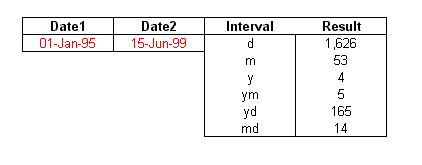The DATEDIF Function

The DATEDIF function is a worksheet function that computes the difference between two dates. This function is available in all versions of Excel since version 5, but is documented only in the help files for Excel 2000.  It isn't documented in either Excel 97 or Excel 2002.  DATEDIF has, for whatever reason, been treated as one of the drunk cousins of the Function Family.  Excel knows he lives a happy and useful existence, and will acknowledge his existence when you ask, but will never mention him in "polite" conversation.

You can use DATEDIF as you would any normal worksheet function, because it is a normal worksheet function.  Until Excel2000, it was never documented in the help files, but it has been around since at least Excel5.  There has been, from time to time, an article in the Knowledge Base describing  DATEDIF, but as often as not, the article is not available.  Why?  Who knows?  One of the deep dark secrets known only the Softies.

The syntax for DATEDIF is as follows:

=DATEDIF(Date1,Date2,Interval)

Where

Date1 is the first date, in standard Excel serial-date format.
Date2 is the second date, in standard Excel serial-date format.
Interval indicates the unit of time that =DATEDIF is to return is result.

Date1 must be less than (earlier) or equal to Date2.   Otherwise, =DATEDIF will return a #NUM! error.  If either Date1 or Date2 is not a date, =DATEDIF will return a #VALUE! error.

Interval must be one of the following codes:

 Code Meaning Description "m" Months The number of complete months between Date1 and Date2. "d Days The number of days between Date1 and Date2. "y" Years The number of complete years between Date1 and Date2. "ym" Months Excluding Year The number of months between Date1 and Date2, as if Date1 and Date2 were in the same year. "yd" Days Excluding Years The number of days between Date1 and Date2, as if Date1 and Date2 were in the same year. "md" Days Excluding Months And Years The number of days between Date1 and Date2, as if Date1 and Date2 were in the same month and the same year.

When passing the interval code to the DATEDIF function, enclose it in quotes if you are passing a literal value to the function:

=DATEDIF(A1,NOW(),"d")

However, if your interval code is stored in a worksheet cell, it should not be enclosed in quotes in the cell.

The table on the right displays the results of the various interval codes for two dates. Pay attention to the result returned by the ym interval code.  The 5 indicates that there are 5 calendar months between January and June -- the years are ignored.  The same is true with the md interval.  There are 14 calendar days between the two dates when the months and years are ignored.Calculating A Person's Age

A frequent use of the DATEDIF is to compute someone's age based on the current date and their birthday. The formula below will return someone's exact age based on their birthday in cell A1.

=DATEDIF(A1,NOW(),"y") & " years, " & DATEDIF(A1,NOW(),"ym") & " months, " & DATEDIF(A1,NOW(),"md") & " days"

This will return a string like

33 years, 9 months, 18 days

You can't use DATEDIF  in VBA code.  VBA provides a function called DateDiff (note, two f's), but DateDiff doesn't support the "ym", "md", and "yd" interval arguments that DATEDIF  does.  To compute age in VBA, you have to do the math on your own.

Function Age(Date1 As Date, Date2 As Date) As String
Dim Y As Integer
Dim M As Integer
Dim D As Integer
Dim Temp1 As Date
Temp1 = DateSerial(Year(Date2), Month(Date1), Day(Date1))
Y = Year(Date2) - Year(Date1) + (Temp1 > Date2)
M = Month(Date2) - Month(Date1) - (12 * (Temp1 > Date2))
D = Day(Date2) - Day(Date1)
If D < 0 Then
M = M - 1
D = Day(DateSerial(Year(date2), Month(date2), 0)) + D
End If
Age = Y & " years " & M & " months " & D & " days"
End Function

DATEDIF And Leap Years

When you are using the yd interval, Excel will use the year of the first date, Date1, to determine whether the to include 29-February in the calculation.  In other words, it uses the year of Date1 as the year for both dates.  For example, if Date1 is 1-Feb-1996, and Date2 is 1-Mar-1997,

=DATEDIF(Date1,Date2,"yd")

will return 29, because there are 29 days between 1-Feb-1996 and 1-Mar-1996.  If Date1 is changed to 1-Feb-1997 and Date2 remains 1-Mar-1997, the same function will return 28, the number of days between 1-Feb-1997 and 1-Mar-1997.   Similarly, if Date1 is 1-Feb-1995 and Date2 is 1-Mar-1996, the  result is 28 because both dates are given the year of the first date, which, in these last two examples, is not a leap year.

 Other Date And Time Related Procedures are described on the following pages. Adding Months And Years The DATEDIF Function Date Intervals Dates And Times Date And Time Entry Date And Time Arithmetic Distributing Dates Over Intervals Holidays Julian Dates Latitude And Longitude  Overtime Hours And Timesheets VBA Procedures For Dates And Times Week Numbers Worksheet Functions For Dates And Times See the Dates And Times Topic Index For Information